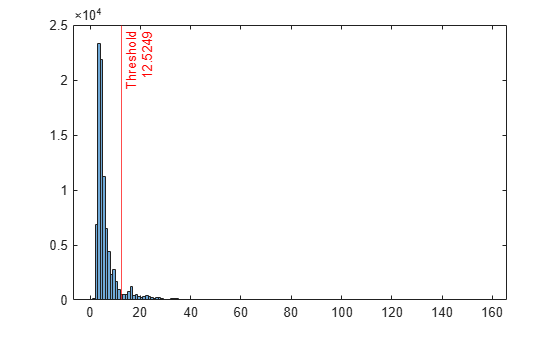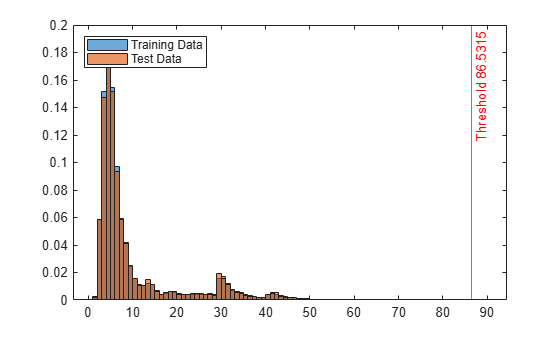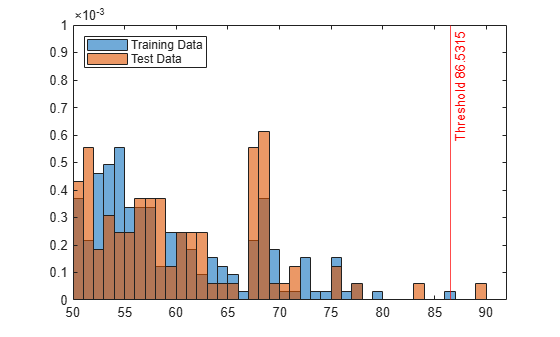# RobustRandomCutForest

Robust random cut forest model for anomaly detection

Since R2023a

## Description

Use a robust random cut forest model object `RobustRandomCutForest` for outlier detection and novelty detection.

• Outlier detection (detecting anomalies in training data) — Detect anomalies in training data by using the `rrcforest` function. The `rrcforest` function returns a `RobustRandomCutForest` model object, anomaly indicators, and scores for the training data.

• Novelty detection (detecting anomalies in new data with uncontaminated training data) — Create a `RobustRandomCutForest` model object by passing uncontaminated training data (data with no outliers) to `rrcforest`. Detect anomalies in new data by passing the object and the new data to the object function `isanomaly`. The `isanomaly` function returns anomaly indicators and scores for the new data.

## Creation

Create a `RobustRandomCutForest` model object by using the `rrcforest` function.

## Properties

expand all

Categorical predictor indices, specified as a vector of positive integers. `CategoricalPredictors` contains index values indicating that the corresponding predictors are categorical. The index values are between 1 and `p`, where `p` is the number of predictors used to train the model. If none of the predictors are categorical, then this property is empty (`[]`).

Collusive displacement calculation method, specified as `'maximal'` or `'average'`.

The software finds the maximum change (`'maximal'`) or the average change (`'average'`) in model complexity for each tree, and computes the collusive displacement (anomaly score) for each observation. For details, see Anomaly Scores.

Fraction of anomalies in the training data, specified as a numeric scalar in the range [0,1].

• If the `ContaminationFraction` value is 0, then `rrcforest` treats all training observations as normal observations, and sets the score threshold (`ScoreThreshold` property value) to the maximum anomaly score value of the training data.

• If the `ContaminationFraction` value is in the range (0,1], then `rrcforest` determines the threshold value (`ScoreThreshold` property value) so that the function detects the specified fraction of training observations as anomalies.

Predictor means of the training data, specified as a numeric vector.

• If you specify `StandardizeData=true` when you train a robust random cut forest model using `rrcforest`:

• The `rrcforest` function does not standardize columns that contain categorical variables. The elements in `Mu` for categorical variables contain `NaN` values.

• The `isanomaly` function standardizes the input data by using the predictor means in `Mu` and standard deviations in `Sigma`.

The length of `Mu` is equal to the number of predictors.

• If you set `StandardizeData=false`, then `Mu` is an empty vector (`[]`).

Number of robust random cut trees (trees in the robust random cut forest model), specified as a positive integer scalar.

Number of observations to draw from the training data without replacement for each robust random cut tree (tree in the robust random cut forest model), specified as a positive integer scalar.

Predictor variable names, specified as a cell array of character vectors. The order of the elements in `PredictorNames` corresponds to the order in which the predictor names appear in the training data.

Threshold for the anomaly score used to identify anomalies in the training data, specified as a numeric scalar in the range [0,`Inf`).

The software identifies observations with anomaly scores above the threshold as anomalies.

Predictor standard deviations of the training data, specified as a numeric vector.

• If you specify `StandardizeData=true` when you train a robust random cut forest model using `rrcforest`:

• The `rrcforest` function does not standardize columns that contain categorical variables. The elements in `Sigma` for categorical variables contain `NaN` values.

• The `isanomaly` function standardizes the input data by using the predictor means in `Mu` and standard deviations in `Sigma`.

The length of `Sigma` is equal to the number of predictors.

• If you set `StandardizeData=false`, then `Sigma` is an empty vector (`[]`).

## Object Functions

 `isanomaly` Find anomalies in data using robust random cut forest

## Examples

collapse all

Detect outliers (anomalies in training data) by using the `rrcforest` function.

Load the sample data set `NYCHousing2015`.

`load NYCHousing2015`

The data set includes 10 variables with information on the sales of properties in New York City in 2015. Display a summary of the data set.

`summary(NYCHousing2015)`
```Variables: BOROUGH: 91446x1 double Values: Min 1 Median 3 Max 5 NEIGHBORHOOD: 91446x1 cell array of character vectors BUILDINGCLASSCATEGORY: 91446x1 cell array of character vectors RESIDENTIALUNITS: 91446x1 double Values: Min 0 Median 1 Max 8759 COMMERCIALUNITS: 91446x1 double Values: Min 0 Median 0 Max 612 LANDSQUAREFEET: 91446x1 double Values: Min 0 Median 1700 Max 2.9306e+07 GROSSSQUAREFEET: 91446x1 double Values: Min 0 Median 1056 Max 8.9422e+06 YEARBUILT: 91446x1 double Values: Min 0 Median 1939 Max 2016 SALEPRICE: 91446x1 double Values: Min 0 Median 3.3333e+05 Max 4.1111e+09 SALEDATE: 91446x1 datetime Values: Min 01-Jan-2015 Median 09-Jul-2015 Max 31-Dec-2015 ```

The `SALEDATE` column is a `datetime` array, which is not supported by `rrcforest`. Create columns for the month and day numbers of the `datetime` values, and then delete the `SALEDATE` column.

```[~,NYCHousing2015.MM,NYCHousing2015.DD] = ymd(NYCHousing2015.SALEDATE); NYCHousing2015.SALEDATE = [];```

The columns `BOROUGH`, `NEIGHBORHOOD`, and `BUILDINGCLASSCATEGORY` contain categorical predictors. Display the number of categories for the categorical predictors.

`length(unique(NYCHousing2015.BOROUGH))`
```ans = 5 ```
`length(unique(NYCHousing2015.NEIGHBORHOOD))`
```ans = 254 ```
`length(unique(NYCHousing2015.BUILDINGCLASSCATEGORY))`
```ans = 48 ```

For a categorical variable with more than 64 categories, the `rrcforest` function uses an approximate splitting method that can reduce the accuracy of the robust random cut forest model. Remove the `NEIGHBORHOOD` column, which contains a categorical variable with 254 categories.

`NYCHousing2015.NEIGHBORHOOD = [];`

Train a robust random cut forest model for `NYCHousing2015`. Specify the fraction of anomalies in the training observations as 0.1, and specify the first variable (`BOROUGH`) as a categorical predictor. The first variable is a numeric array, so `rrcforest` assumes it is a continuous variable unless you specify the variable as a categorical variable.

```rng("default") % For reproducibility [Mdl,tf,scores] = rrcforest(NYCHousing2015, ... ContaminationFraction=0.1,CategoricalPredictors=1);```

`Mdl` is a `RobustRandomCutForest` model object. `rrcforest` also returns the anomaly indicators (`tf`) and anomaly scores (`scores`) for the training data `NYCHousing2015`.

Plot a histogram of the score values. Create a vertical line at the score threshold corresponding to the specified fraction.

```histogram(scores) xline(Mdl.ScoreThreshold,"r-",["Threshold" Mdl.ScoreThreshold])```If you want to identify anomalies with a different contamination fraction (for example, 0.01), you can train a new robust random cut forest model.

```rng("default") % For reproducibility [newMdl,newtf,scores] = rrcforest(NYCHousing2015, ... ContaminationFraction=0.01,CategoricalPredictors=1); ```

If you want to identify anomalies with a different score threshold value (for example, 65), you can pass the `RobustRandomCutForest` model object, the training data, and a new threshold value to the `isanomaly` function.

```[newtf,scores] = isanomaly(Mdl,NYCHousing2015,ScoreThreshold=65); ```

Note that changing the contamination fraction or score threshold changes the anomaly indicators only, and does not affect the anomaly scores. Therefore, if you do not want to compute the anomaly scores again by using `rrcforest` or `isanomaly`, you can obtain a new anomaly indicator using the existing score values.

Change the fraction of anomalies in the training data to `0.01`.

`newContaminationFraction = 0.01;`

Find a new score threshold by using the `quantile` function.

`newScoreThreshold = quantile(scores,1-newContaminationFraction)`
```newScoreThreshold = 63.2642 ```

Obtain a new anomaly indicator.

`newtf = scores > newScoreThreshold;`

Create a `RobustRandomCutForest` model object for uncontaminated training observations by using the `rrcforest` function. Then detect novelties (anomalies in new data) by passing the object and the new data to the object function `isanomaly`.

Load the 1994 census data stored in `census1994.mat`. The data set contains demographic data from the US Census Bureau to predict whether an individual makes over \$50,000 per year.

`load census1994`

`census1994` contains the training data set `adultdata` and the test data set `adulttest`.

Assume that `adultdata` does not contain outliers. Train a robust random cut forest model for `adultdata`. Specify `StandardizeData` as `true` to standardize the input data.

```rng("default") % For reproducibility [Mdl,tf,s] = rrcforest(adultdata,StandardizeData=true);```

`Mdl` is a `RobustRandomCutForest` model object. `rrcforest` also returns the anomaly indicators `tf` and anomaly scores `s` for the training data `adultdata`. If you do not specify the `ContaminationFraction` name-value argument as a value greater than 0, then `rrcforest` treats all training observations as normal observations, meaning all the values in `tf` are logical 0 (`false`). The function sets the score threshold to the maximum score value. Display the threshold value.

`Mdl.ScoreThreshold`
```ans = 86.5315 ```

Find anomalies in `adulttest` by using the trained robust random cut forest model. Because you specified `StandardizeData=true` when you trained the model, the `isanomaly` function standardizes the input data by using the predictor means and standard deviations of the training data stored in the `Mu` and `Sigma` properties, respectively.

`[tf_test,s_test] = isanomaly(Mdl,adulttest);`

The `isanomaly` function returns the anomaly indicators `tf_test` and scores `s_test` for `adulttest`. By default, `isanomaly` identifies observations with scores above the threshold (`Mdl.ScoreThreshold`) as anomalies.

Create histograms for the anomaly scores `s` and `s_test`. Create a vertical line at the threshold of the anomaly scores.

```histogram(s,Normalization="probability") hold on histogram(s_test,Normalization="probability") xline(Mdl.ScoreThreshold,"r-",join(["Threshold" Mdl.ScoreThreshold])) legend("Training Data","Test Data",Location="northwest") hold off```Display the observation index of the anomalies in the test data.

`find(tf_test)`
```ans = 3541 ```

The anomaly score distribution of the test data is similar to that of the training data, so `isanomaly` detects a small number of anomalies in the test data with the default threshold value.

Zoom in to see the anomaly and the observations near the threshold.

```xlim([50 92]) ylim([0 0.001])```You can specify a different threshold value by using the `ScoreThreshold` name-value argument. For an example, see Specify Anomaly Score Threshold.